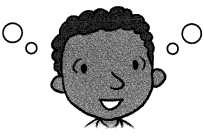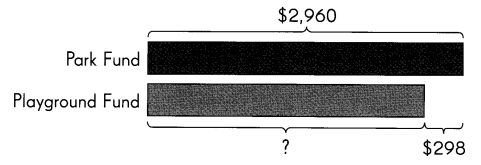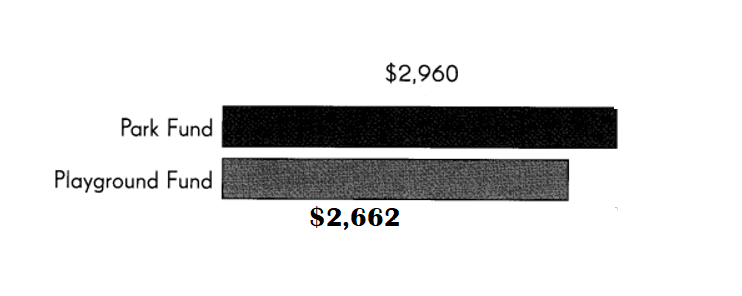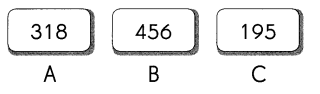Go through the Math in Focus Grade 3 Workbook Answer Key Chapter 5 Using Bar Models: Addition and Subtraction to finish your assignments.

Math Journal

The Park Fund raises $2,960. The Playground Fund raises$2,662.Example
Word problem
The Park Fund raises $2,960. The Playground Fund raises$298 less than the Park Fund. How much does the Playground Fund raise?
ModelSolution
$2,960 –$298 = $2,662 The Playground Fund raises$2,662.

Now you try it!
Word problem
The Park Fund raises $2,960. The Playground Fund raises$2,662. Who raised more funds and how much.
ModelSolution:
Park funds raised $298 more than playground funds. Explanation: Amount of funds park raised =$2960.
Amount of funds playground raised = $2662. Difference: Amount of funds park raised – Amount of funds playground raised =$2960 – $2662 =$298.

Challenging Practice
Look at the cards.Think of three ways to choose two cards. Find the sum of the two cards.
A and B ; 318 + 456 = 774

Question 1.
____ and ___ ; ____ + ____ = ____
B and C ; 456 + 195 = 651.

Explanation:
B + C ; 456 + 195
= 651.

Question 2.
____ and ___ ; ____ + ____ = ____
C and A ; 195 + 318 = 513.

Explanation:
C + A ; 195 + 318
= 513.

Fill in the missing letters.
Question 3.
Which two cards give the greatest sum? ____ and ________
A and B two cards give the greatest sum of 774.

Explanation:
A and B ; 318 + 456 = 774
B and C ; 456 + 195 = 651.
C and A ; 195 + 318 = 513.

Question 4.
Which two cards give the least sum? ____ and __________
C and A two cards give the least sum.

Explanation:
A and B ; 318 + 456 = 774
B and C ; 456 + 195 = 651.
C and A ; 195 + 318 = 513.

Question 5.
Which two cards give the greatest difference? __________ and __________
B and C two cards give the greatest difference.

Explanation:
A = 318.
B = 456.
C = 195.
Difference:
B – A = 456 – 318 = 138.
B – C = 456 – 195 = 261.
A – C = 318 – 195 = 123.

Question 6.
Which two cards give the least difference? ___________ and ___________
A and C two cards give the least difference.

Explanation:
A = 318.
B = 456.
C = 195.
Difference:
B – A = 456 – 318 = 138.
B – C = 456 – 195 = 261.
A – C = 318 – 195 = 123.

Problem Solving
Question 1.
Carlos has been collecting cards since he was 5 years old. He has not thrown away any of his cards. He is now 7 years old. He collected 201 cards last year. He collects 1 25 cards this year. He has a total of 589 cards now.
a. How many cards did he have in total at the end of last year?
201 cards he has in total at the end of last year.

Explanation:
Number of cards he collects in last years = 201.
Number of cards he collects in this years = 125.
Total number of cards he has now = 589.

b. How many cards did he collect when he was 5 years old?
Total number of cards he collected when he was 5 years old = 263.

Explanation:
Number of cards he collects in last year = 201.
Number of cards he collects in this year = 125.
Total number of cards he has now = 589.
Total number of cards he collected when he was 5 years old = Total number of cards he has now  – ( Number of cards he collects in last years + Number of cards he collects in this year )
= 589 – (201 + 125)
= 589 – 326
= 263.

Question 2.
Jason, Peter, and Ken hold a garage sale for charity. Jason raises $350. Peter raises$20 more than Jason. Ken raises the same amount as the total amount raised by Jason and Peter. How much money do the three boys raise in all?
Amount of money the three boys raise in all = $1440. Explanation: Amount of money Jason raises =$350.
Peter raises $20 more than Jason. => Amount of money Peter raises =Amount of money Jason raises +$20
=> $350 +$20
= $370. Ken raises the same amount as the total amount raised by Jason and Peter. => Amount of money Ken raises = Amount of money Jason raises + Amount of money Peter raises =>$350 + $370 =$ 720.
= $350 +$370 + $720 =$720 + $720 =$1440.